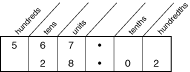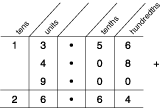Przejdź do głównych treści Przejdź do pola wyszukiwania
Summary Article: decimal number system
From The Hutchinson Unabridged Encyclopedia with Atlas and Weather Guide

Most commonly used number system, to the base ten. Decimal numbers do not necessarily contain a decimal point; 563, 5.63, and −563 are all decimal numbers. Other systems are mainly used in computing and include the binary number system, octal number system, and hexadecimal number system.

The decimals 0.3, 0.51, and 0.023 can be expressed as the decimal fractions3/10, 51/100, and 23/1,000. They are all terminating decimals. These fractions can equally be expressed as the percentages 30%, 51%, and 2.3%.

Decimal numbers may be thought of as written under column headings based on the number 10. For example:Using the table, 567 stands for 5 hundreds, 6 tens, and 7 units; 28.02 stands for 2 tens, 8 units, and 2 hundredths.

567 has no numbers after the decimal point, that is 0 decimal places. 28.02 has 2 numbers after the decimal point, that is 2 decimal places.

Large decimal numbers may also be expressed in floating-point notation.

Addition and subtraction When adding or subtracting decimals it is important to keep the decimal points underneath each other. For example, to work out 13.56 + 4.08 + 9:Using the addition table, the answer is 26.64.

essays

Working out percentage increases

Expressing a recurring decimal as a fraction

Calculating with fractions, decimals and percentages

Calculating powers and square roots

Calculating with rounding and multiples

Place value, sum and difference

Pie charts

Calculating with decimals

A Plus Maths

CoolMath

Maths Year 2000

Decimals, Whole Numbers, and Exponents

Discovery School's Webmath

Easymaths

Fractions

Funbrain

Interactive Mathematics: Miscellany and Puzzles

Mathematics Lessons that Are Fun, Fun, Fun!

MATHguide

Maths Is Fun

Maths Online

Plus Magazine

S-Cool! GCSE Maths Revision Guide© RM, 2018. All rights reserved.

Pokrewne wpisy w Credo

Full text Article Decimal system
Illustrated Dictionary of Science, Andromeda

A number system using the powers of ten; our everyday system of numeration. The digits used are 0, 1, 2, 3, 4, 5, 6, 7, 8, 9; the powers of 10...

Full text Article decimal system
The Macmillan Encyclopedia

The number system in common use, having a base 10 and thus using ten separate numerals. It also involves the use of a decimal point to express...

Full text Article Number systems
50 Maths Ideas You Really Need to Know

A number system is a method for handling the concept of ‘how many’. Different cultures at differing periods of time have adopted various methods, ra

Patrz więcej wyników Credo